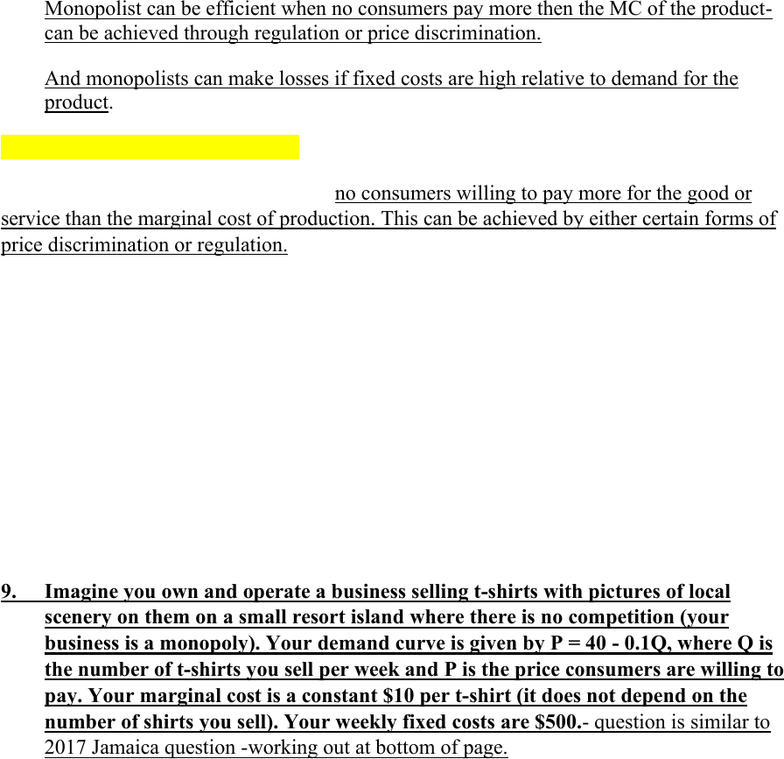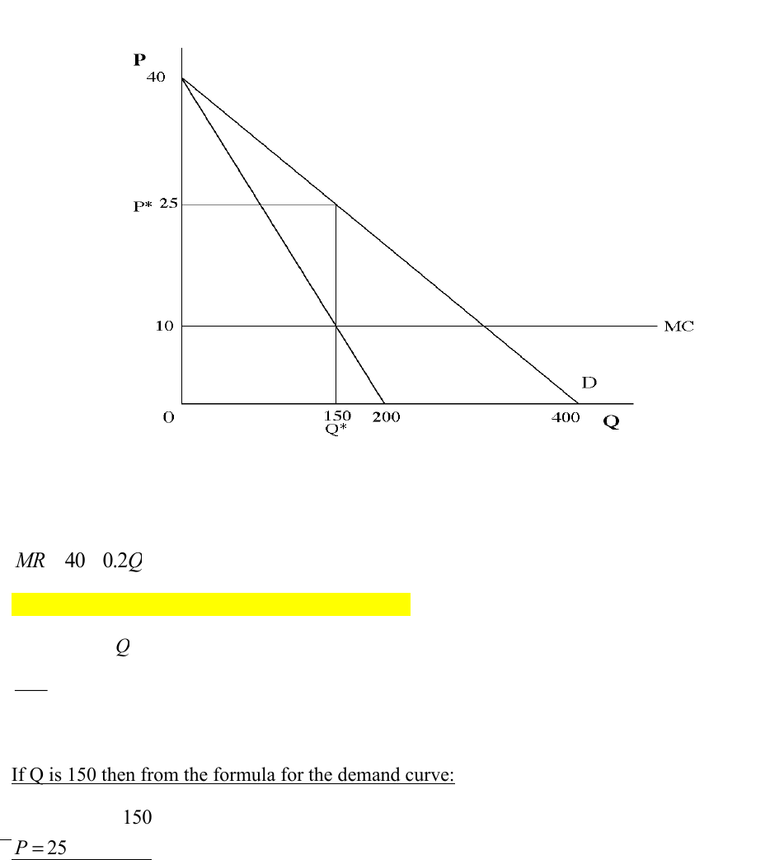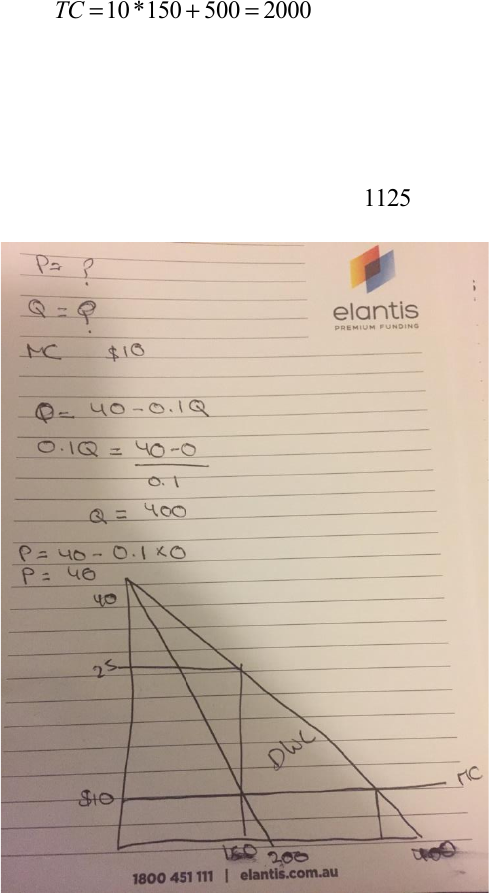# ECON1001 Study Guide - Final Guide: Price Discrimination, Demand Curve, Marginal Revenue

51 views9 pages
School
Department
Course
Professor2. Do you agree with the following statement? “Monopolists always earn excessive
The statement is incorrect. Monopolists often earn excessive profits and are inefficient
but not always. [2 points]
Regulation or more sophisticated pricing can address these problems. [3 points].
Monopolist can be efficient when no consumers pay more then the MC of the product-
can be achieved through regulation or price discrimination.
And monopolists can make losses if fixed costs are high relative to demand for the
product. [3 points]
1. When is monopoly efficient?
A monopoly is efficient when there are no consumers willing to pay more for the good or
service than the marginal cost of production. This can be achieved by either certain forms of
price discrimination or regulation.
2. Explain how economic forces drive economic profits to zero in the long run in
perfect competition.
If there are economic profits in an industry firms will enter the industry. This shifts the
supply curve to the right and as long as demand is downward sloping prices fall. This reduces
the gap between ATC and price and therefore, economic profit. Firms will continue entering
until P=ATC and so there is no economic profit. If P<ATC then economic profit is negative
and firms will exit, shifting the supply curve left and prices up until P=ATC.
9. Imagine you own and operate a business selling t-shirts with pictures of local
scenery on them on a small resort island where there is no competition (your
business is a monopoly). Your demand curve is given by P = 40 - 0.1Q, where Q is
the number of t-shirts you sell per week and P is the price consumers are willing to
pay. Your marginal cost is a constant \$10 per t-shirt (it does not depend on the
number of shirts you sell). Your weekly fixed costs are \$500.- question is similar to
2017 Jamaica question -working out at bottom of page.
a. Draw a graph of your demand and marginal cost curves.
b. Write down the formula for your marginal revenue curve and add the curve to the
graph.
c. Calculate the profit maximizing price and quantity and show them on your graph
as well as showing in writing how you found them.
Unlock document

This preview shows pages 1-3 of the document.
Unlock all 9 pages and 3 million more documents.d. Calculate your economic profit. Hint: Note that marginal cost is equal to average
variable cost in this case.
e. Calculate the deadweight loss due to the monopoly.
[3 points for each correct answer]
a.
b.
MR =40 -0.2Q
c. For the profit maximizing quantity, MR = MC:
10 =40 -0.2Q
-30
-.2 =Q
Q=150
If Q is 150 then from the formula for the demand curve:
P=40 -0.1*150
P=25
d. Economic profit is revenue total costs. With 150 shirts at \$25 each, revenue is \$3,750.
Unlock document

This preview shows pages 1-3 of the document.
Unlock all 9 pages and 3 million more documents.TC =MC *Q+FC
TC =10 *150 +500 =2000
Therefore profit is \$1,750.
e. The deadweight loss is the triangle under the demand curve to the right of the level of
sales under monopoly, which is \$1,125.
DL =25 -10
( )
*(300 -150) /2 =1125
Unlock document

This preview shows pages 1-3 of the document.
Unlock all 9 pages and 3 million more documents.